# PSAT Math : How to use FOIL

## Example Questions

### Example Question #1 : How To Use Foil

Factor 2x2 - 5x – 12

(x - 4) (2x + 3)

(x + 4) (2x + 3)

(x – 4) (2x – 3)

(x + 4) (2x + 3)

(x - 4) (2x + 3)

Explanation:

Via the FOIL method, we can attest that x(2x) + x(3) + –4(2x) + –4(3) = 2x2 – 5x – 12.

### Example Question #2 : How To Use Foil

x > 0.

Quantity A: (x+3)(x-5)(x)

Quantity B: (x-3)(x-1)(x+3)

Quantity B is greater

Quantity A is greater

The relationship cannot be determined from the information given

The two quantities are equal

Quantity B is greater

Explanation:

Use FOIL:

(x+3)(x-5)(x) = (x2 - 5x + 3x - 15)(x) = x3 - 5x2 + 3x2 - 15x = x3 - 2x2 - 15x for A.

(x-3)(x-1)(x+3) = (x-3)(x+3)(x-1) = (x2 + 3x - 3x - 9)(x-1) = (x2 - 9)(x-1)

(x2 - 9)(x-1) = x3 - x2 - 9x + 9 for B.

The difference between A and B:

(x3 - 2x2 - 15x) - (x3 - x2 - 9x + 9) = x3 - 2x2 - 15x - x3 + x2 + 9x - 9

= - x2 - 4x - 9. Since all of the terms are negative and x > 0:

A - B < 0.

Rearrange A - B < 0:

A < B

### Example Question #3 : How To Use Foil

Solve for all real values of.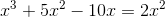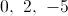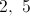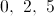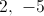Explanation: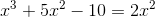First, move all terms to one side of the equation to set them equal to zero.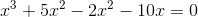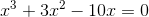All terms contain an, so we can factor it out of the equation.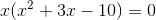Now, we can factor the quadratic in parenthesis. We need two numbers that add toand multiply to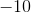.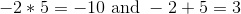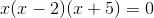We now have three terms that multiply to equal zero. One of these terms must equal zero in order for the product to be zero.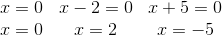Our answer will be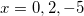.

### Example Question #1 : Exponents And The Distributive Property

Simplify: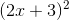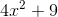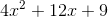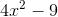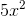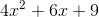Explanation:

In order to simplify this expression, you need to use the FOIL method. First rewrite the expression to look like this: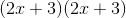Next, multiply your first terms together: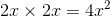Then, multiply your outside terms together: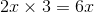Then, multiply your inside terms together: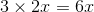Lastly, multiply your last terms together: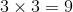Together, you have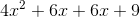You can combine your like terms,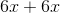, to give you the final answer:### Example Question #2 : Exponents And The Distributive Property

Use FOIL to simplify the following product: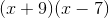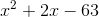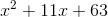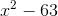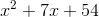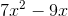Explanation:

Use the FOIL method (first, outside, inside, last) to find the product of:First: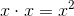Outside: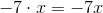Inside: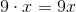Last: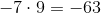Sum the products to find the polynomial: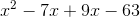### Example Question #3 : Exponents And The Distributive Property

Simplify: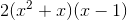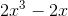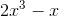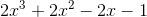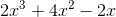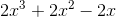Explanation:

To solve this problem, use the FOIL method. Start by multiplying the First term in each set of parentheses: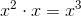Then multiply the outside terms: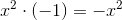Next, multiply the inside terms:Finally, multiply the last terms: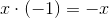When you put the pieces together, you have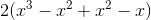. Notice that the middle terms cancel each other out, and you are left with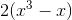. When you distribute the two, you reach the answer: